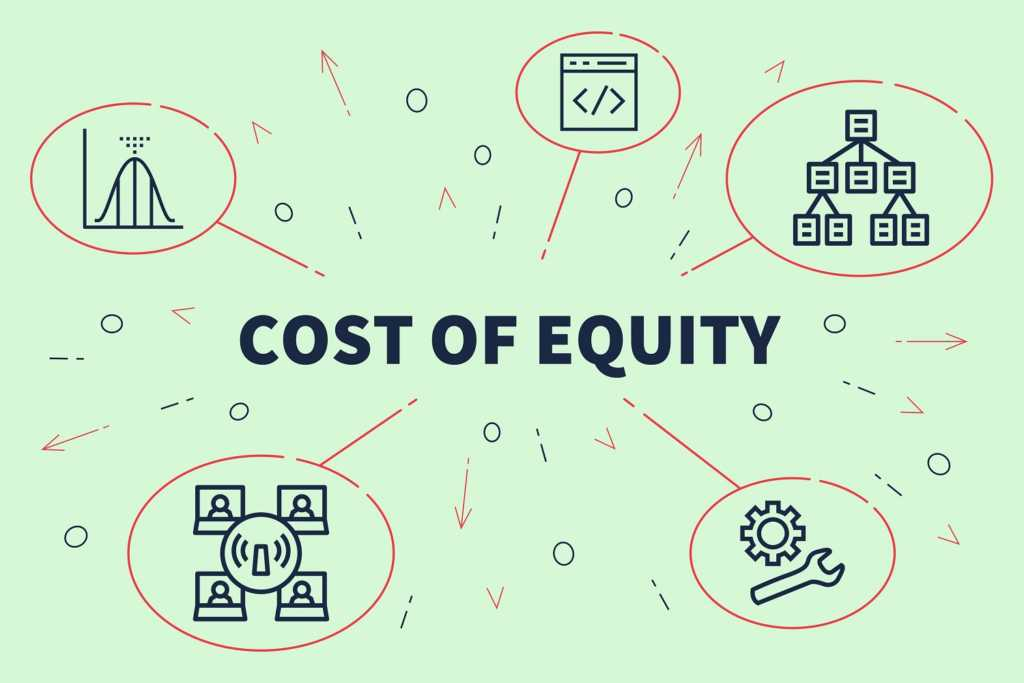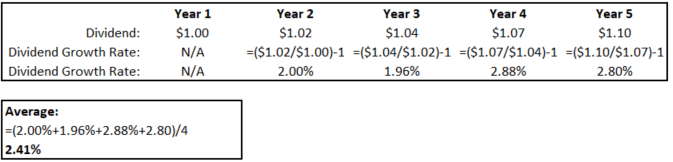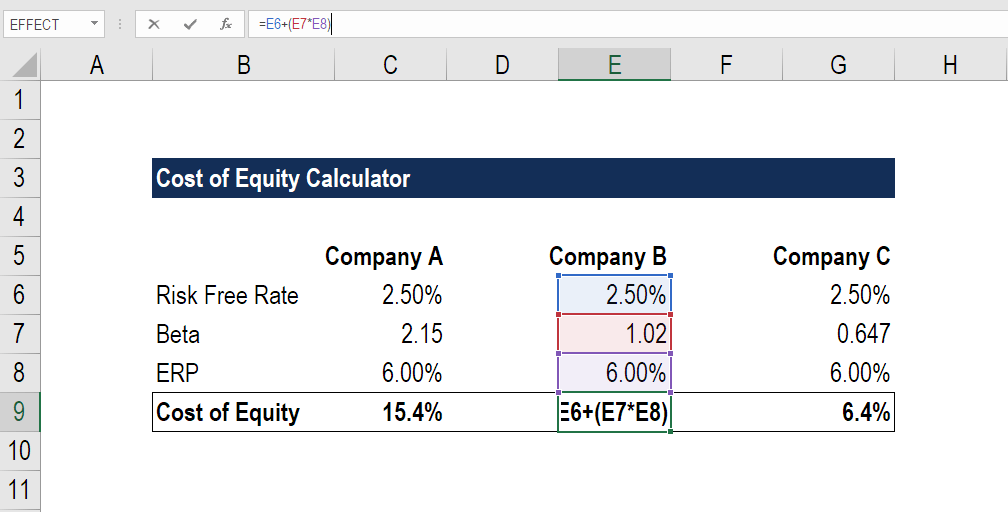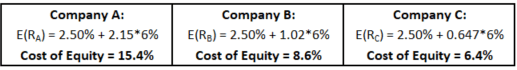# Cost of Equity

The rate of return a shareholder requires for investing equity

## What is Cost of Equity?

Cost of Equity is the rate of return a shareholder requires for investing equity into a business. The rate of return an investor requires is based on the level of risk associated with the investment, which is measured as the historical volatility of returns. A firm uses cost of equity to assess the relative attractiveness of investments, including both internal projects and external acquisition opportunities. Companies typically use a combination of equity and debt financing, with equity capital being more expensive.### How to Calculate Cost of Equity

The cost of equity can be calculated by using the CAPM (Capital Asset Pricing Model) or Dividend Capitalization Model (for companies that pay out dividends).

#### 1. CAPM (Capital Asset Pricing Model)

CAPM takes into account the riskiness of an investment relative to the market. The model is less exact due to the estimates made in the calculation (because it uses historical information).

CAPM Formula:

E(Ri) = Rf + β* [E(Rm) – Rf]

Where:

E(Ri) = Expected return on asset i

Rf = Risk-free rate of return

βi = Beta of asset i

E(Rm) = Expected market return

##### Risk-Free Rate of Return

The return expected from a risk-free investment (if computing the expected return for a US company, the 10-year Treasury note could be used).

##### Beta

The measure of systematic risk (the volatility) of the asset relative to the market. Beta can be found online or calculated by using regression: dividing the covariance of the asset and market’s returns by the variance of the market.

βi < 1: Asset i is less volatile (relative to the market)

βi = 1: Asset i’s volatility is the same rate as the market

βi > 1: Asset i is more volatile (relative to the market)

##### Expected Market Return

This value is typically the average return of the market (which the underlying security is a part of) over a specified period of time (five to ten years is an appropriate range).

##### Example of CAPM

Chris is looking to invest in XYZ Co. (a US company) and would like to figure out his expected return on the security. The yield of a 10-year Treasury note is 2.21%, the beta of XYZ Co. is 1.34 and the average return of the S&P 500 over the past 10 years is 7%. What is the expected return per share?

• Rf = 2.21%
• βi = 1.34
• E(Rm) = 7%

E(Ri) = 2.21% + 1.34*(7% – 2.21%)

E(Ri) = 8.6286%

Chris’s expected return on a share of XYZ Co. is 8.6286%.

#### 2. Dividend Capitalization Model

The Dividend Capitalization Model only applies to companies that pay dividends, and it also assumes that the dividends will grow at a constant rate. The model does not account for investment risk to the extent that CAPM does (since CAPM requires beta).

Dividend Capitalization Formula:

Re = (D1 / P0) + g

Where:

Re = Cost of Equity

D1 = Dividends/share next year

P0 = Current share price

g = Dividend growth rate

##### Dividends/Share Next Year

Companies usually announce dividends far in advance of the distribution. The information can be found in company filings (annual and quarterly reports or through press releases). If the information cannot be located, an assumption can be made (using historical information to dictate whether the next year’s dividend will be similar).

##### Current Share Price

The share price of a company can be found by searching the ticker or company name on the exchange that the stock is being traded on, or by simply using a credible search engine.

##### Dividend Growth Rate

The Dividend Growth Rate can be obtained by calculating the growth (each year) of the company’s past dividends and then taking the average of the values.

The growth rate for each year can be found by using the following equation:

Dividend Growth = (Dt/Dt-1) – 1

Where:

Dt = Dividend payment of year t

Dt-1 = Dividend payment of year t-1 (one year before year t)

##### Example

Below are the dividend amounts paid every year by a company that has been operating for five years.The average of the growth rates is 2.41%.

### Dividend Capitalization Model Example

XYZ Co. is currently being traded at \$5 per share and just announced a dividend of \$0.50 per share, which will be paid out next year. Using historical information, an analyst estimated the dividend growth rate of XYZ Co. to be 2%. What is the cost of equity?

• D1 = \$0.50
• P0 = \$5
• g = 2%

Re = (\$0.50/\$5) + 2%

Re = 12%

The cost of equity for XYZ Co. is 12%.

### Cost of Equity Example in Excel (CAPM Approach)

Step 1: Find the RFR (risk-free rate) of the market

Step 2: Compute or locate the beta of each company

Step 3: Calculate the ERP (Equity Risk Premium)

ERP = E(Rm) – Rf

Where:

E(Rm) = Expected market return

Rf = Risk-free rate of return

Step 4: Use the CAPM formula to calculate the cost of equity.

E(Ri) = Rf + βi*ERP

Where:

E(Ri) = Expected return on asset i

Rf = Risk free rate of return

βi = Beta of asset i

ERP (Equity Risk Premium) = E(Rm) – RfThe company with the highest beta sees the highest cost of equity and vice versa. It makes sense because investors must be compensated with a higher return for the risk of more volatility (a higher beta).

### Cost of Equity vs Cost of Debt

Cost of equity is often higher than Cost of Debt. Equity investors are compensated more generously because equity is riskier than debt, given that:

• Debtholders are paid before equity investors (absolute priority rule).
• Debtholders are guaranteed payments, while equity investors are not.
• Debt is often secured by specific assets of the firm, while equity is not.
• In exchange for taking less risk, debtholders have a lower expected rate of return.

### Cost of Equity vs WACC

The cost of equity applies only to equity investments, whereas the Weighted Average Cost of Capital (WACC) accounts for both equity and debt investments.

Cost of equity can be used to determine the relative cost of an investment if the firm doesn’t possess debt (i.e. the firm only raises money through issuing stock).

The WACC is used instead for a firm with debt. The value will always be cheaper because it takes a weighted average of the equity and debt rates (and debt financing is cheaper).

### Cost of Equity in Financial Modeling

WACC is typically used as a discount rate for unlevered free cash flow (FCFF). Since WACC accounts for the cost of equity and cost of debt, the value can be used to discount the FCFF, which is the entire free cash flow available to the firm. It is important to discount it at the rate it costs to finance (WACC).

Cost of equity can be used as a discount rate if you use levered free cash flow (FCFE). The cost of equity represents the cost to raise capital from equity investors, and since FCFE is the cash available to equity investors, it is the appropriate rate to discount FCFE by.

CFI is a global provider of financial modeling certification programs for aspiring financial analysts working in investment banking, equity research, corporate development, and FP&A.  To continue advancing your career, these additional resources will be helpful:

• Return on Equity
• Enterprise Value
• Market Capitalization
• Valuation Methods

### Financial Analyst Training

Get world-class financial training with CFI’s online certified financial analyst training program!

Gain the confidence you need to move up the ladder in a high powered corporate finance career path.

Learn financial modeling and valuation in Excel the easy way, with step-by-step training.